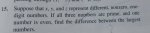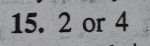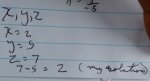# two questions about: Let x,y,z be distinct, non-zero, one-digit numbers.

#### allegansveritatem

##### Full Member
This simple problem is from a series of review problems:Here is the book's solution:Here is what I did:Question 1) Where did the author get the 4? The two largest numbers are 5 and 7 and the difference can only be 2 or -2.

Question 2) Is there a way to solve this problem using algebra? Or is that even expected here?

#### pka

##### Elite Member
This simple problem is from a series of review problems:
View attachment 10597

Here is the book's solution:
View attachment 10598
Here is what I did:
View attachment 10599
Question 1) Where did the author get the 4? The two largest numbers are 5 and 7 and the difference can only be 2 or -2.
Question 2) Is there a way to solve this problem using algebra? Or is that even expected here?
There are four one digit prime numbers: $$\displaystyle 2,~3,~5,~\&~7$$
Read again carefully the wording. Then repost.

#### Dr.Peterson

##### Elite Member
This simple problem is from a series of review problems:
View attachment 10597

Here is the book's solution:
View attachment 10598

Here is what I did:
View attachment 10599

Question 1) Where did the author get the 4? The two largest numbers are 5 and 7 and the difference can only be 2 or -2.

Question 2) Is there a way to solve this problem using algebra? Or is that even expected here?
This is not an algebra problem. I'd say it's just testing general reasoning skills.

You can't assume the numbers are 2, 5, 7. What other possibilities are there for the three numbers?

#### allegansveritatem

##### Full Member
This is not an algebra problem. I'd say it's just testing general reasoning skills.

You can't assume the numbers are 2, 5, 7. What other possibilities are there for the three numbers?
I'm afraid I ASSUMED that the two digits, the ones other than 2, had to be contiguous. Don't ask me why. I suppose it is because there is a category of word problem where we are asked to find contiguous even or odd digits that have such and such addends and/or subtrahends. A good motto when dealing with word problems: Assume nothing!

#### allegansveritatem

##### Full Member
There are four one digit prime numbers: $$\displaystyle 2,~3,~5,~\&~7$$
Read again carefully the wording. Then repost.
I catch your drift. I assumed certain conditions that did not warrant assumption.

#### pka

##### Elite Member
I catch your drift. I assumed certain conditions that did not warrant assumption.
Each of the three numbers is prime & one is even.
Thus the selection is $$\displaystyle \{2,3,5\},~\{2,3,7\},\text{ or }\{2,5,7\}$$

$$\displaystyle 7-3=4,\text{ or }7-5=2$$

Last edited: